# 前端跳槽面试算法——动态规划

16142

Part1改编自《漫画算法》原作者：程序员小灰

## 前言• Part1：通过漫画形象地讲述动态规划的思想
• Part2：配合一道有关动态规划的LeetCode真题进行实战演练

## Part1：漫画理解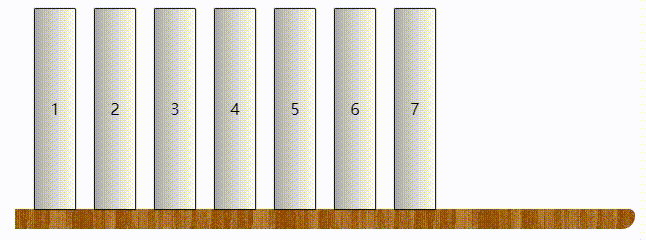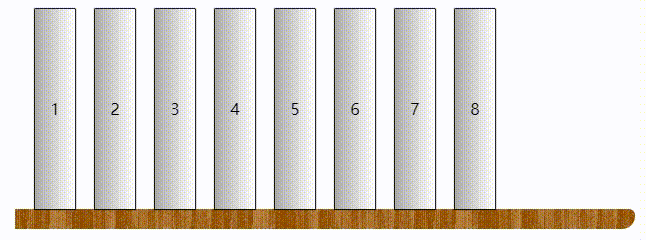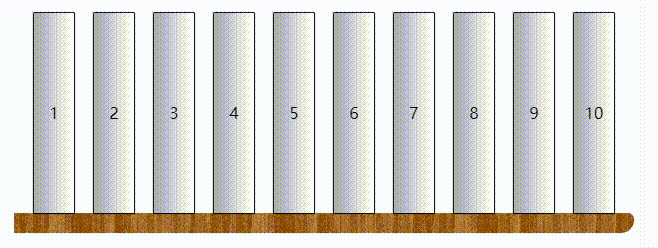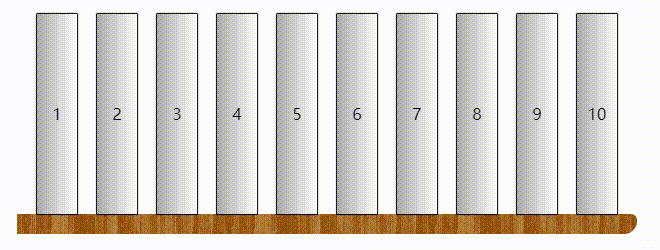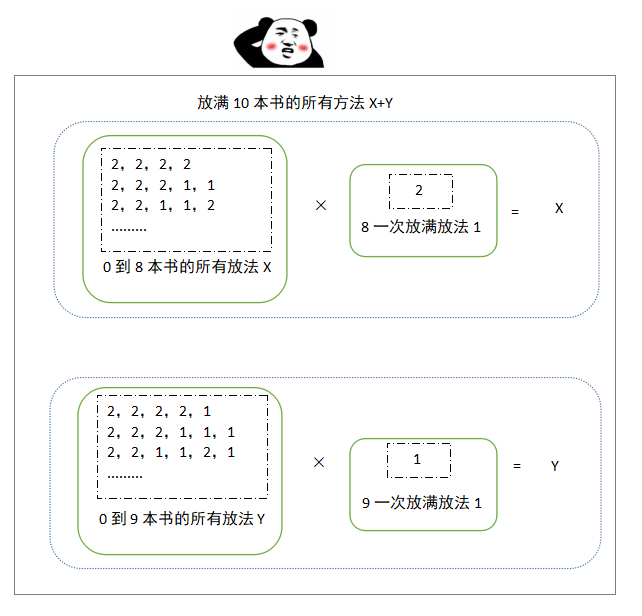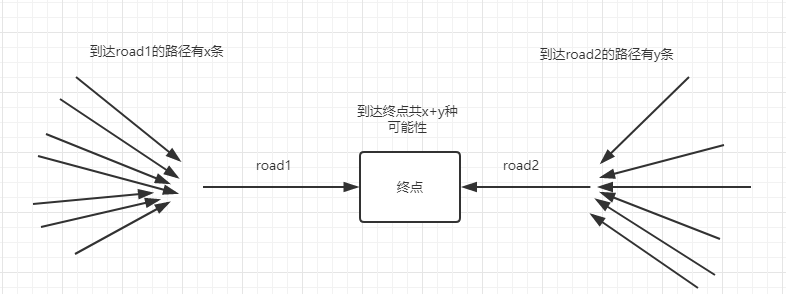(相当于我们把放书的最后一步分为放2本和放1本两种情况)F(1) = 1;

F(2) = 2;

F(n) = F(n-1)+F(n-2)（n>=3）## Part2：实战演练

### 不同路径Ⅱ

LeetCode第63题  原题地址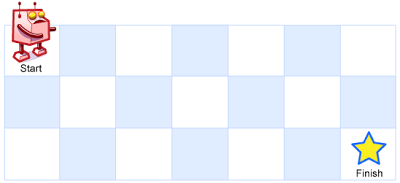[
[0,0,0],
[0,1,0],
[0,0,0]

从左上角到右下角一共有 2 条不同的路径：
1. 向右 -> 向右 -> 向下 -> 向下
2. 向下 -> 向下 -> 向右 -> 向右

### 题目解析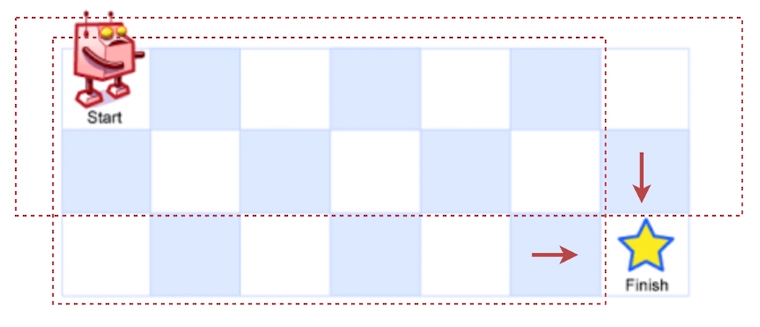7 * 3 矩阵

• 第一种边界F(1*n) 即1行n列• 第二种边界F(n*1) 即n行1列### 代码实现

``````export default (arr) => {
let dp = (m, n) => {
// 检查起始或者目标元素是不是1(障碍物)，如果起始或者最后那个格就是1，说明怎么都怎么不到那，
// 直接返回0
if (arr[m - 1][n - 1] === 1 || arr === 1) {
return 0
}
// 有边界
if (m < 2 || n < 2) {
// 第一种边界 1行n列
if (m < 2) {
return arr[m - 1].includes(1) ? 0 : 1
} else {
// 第二种边界 n行1列
for (let i = 0; i < m; i++) {
if (arr[i] === 1) {
return 0
}
}
return 1
}
} else {
// 递归
return dp(m - 1, n) + dp(m, n - 1)
}
}
return dp(arr.length, arr.length)
}``````

## 补充：时间复杂度分析

### 问题分析

• F(10) = F(9) + F(8)
• F(9) = F(8) + F(7)

•  F(8) + F(8) + F(7)

• F(7) + F(7) +F(7) + F(6) + F(6)

F(n)不单纯是一个值的引用，他是一个递归函数，我们每重复一次它都会重新执行一次F函数

### 提出思路

1. 缓存每一次计算出的值，也就是记录下F(9),F(8),F(7)...的值，每次递归到有之前计算过数据直接拿值，而不用再次重复调用递归函数。
2. 从下向上(由起点至终点)计算，由于每次只依赖前两个数据，通过两个变量只保存前两次的数据就可以了，如计算F(3)只依赖F(1)和F(2)，计算F(6)只依赖F(5)和F(4)。

### 代码优化

``````// 传入二维数组
arr => {
// 行数
let n = arr.length;
if(!n){
return 0;
}
// 列数
let m = arr.length;
// 起点或终点为障碍物
if(arr === 1 || arr[n - 1][m - 1] === 1){
return 0;
}
// 记录到达每个位置的路径可能数
var rode= [];
// 遍历每一行
for(let i = 0; i < n; i++){
rode[i] = [];    // 遍历每一行的每个元素
for(let j = 0; j < m; j++){
// 若某节点是障碍物，则通向该节点的路径数量为0
if(arr[i][j] === 1){
rode[i][j] = 0;
} else if(i === 0){
// 若是第一行 每个节点是否能通过都依赖它左方节点
rode[i][j] = rode[i][j - 1] === 0 ? 0 : 1;
} else if(j === 0){
// 若是第一列 每个节点是否能通过都依赖它上方节点
rode[i][j] = rode[i - 1][j] === 0 ? 0 : 1;
} else {
// 否则递归
rode[i][j] = rode[i - 1][j] + rode[i][j - 1];
}
}
}
return rode[n - 1][m - 1];
}``````

## 总结

•••••••••